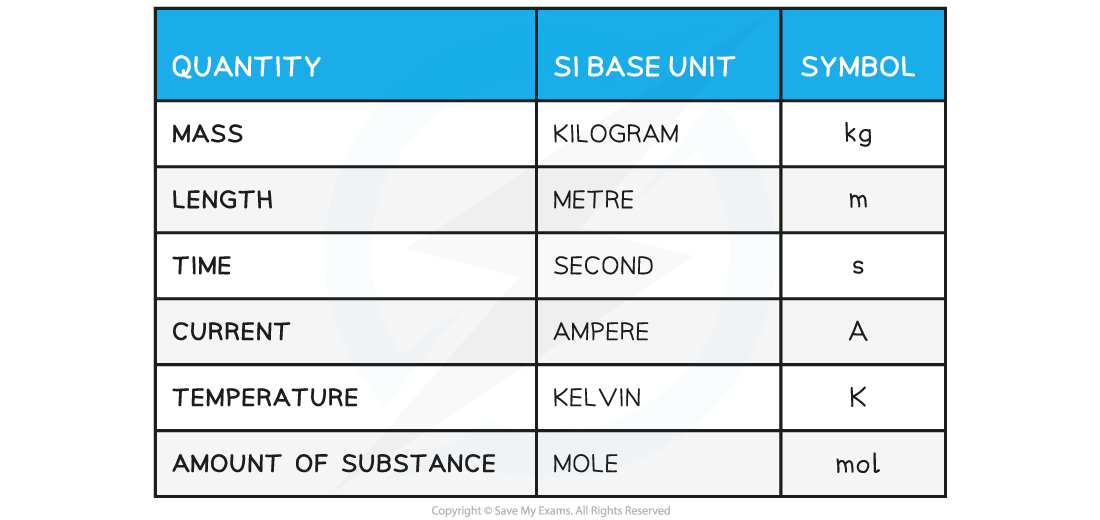# AQA A Level Physics复习笔记1.1.1 SI Units

### SI Base Quantities

• There is a seemingly endless number of units in Physics
• These can all be reduced to six base units from which every other unit can be derived
• These seven units are referred to as the SI Base Units; this is the only system of measurement that is officially used in almost every country around the world

SI Base Quantities Table#### Exam Tip

You will only be required to use the first five SI base units in this course, so make sure you know them!

### Derived Units

• Derived units are derived from the seven SI Base units
• The base units of physical quantities such as:
• Newtons, N
• Joules, J
• Pascals, Pa, can be deduced

• To deduce the base units, it is necessary to use the definition of the quantity
• The Newton (N), the unit of force, is defined by the equation:
• Force = mass × acceleration
• N = kg × m s–2 = kg m s–2
• Therefore, the Newton (N) in SI base units is kg m s–2

• The Joule (J), the unit of energy, is defined by the equation:
• Energy = ½ × mass × velocity2
• J = kg × (m s–1)2 = kg m2 s–2
• Therefore, the Joule (J) in SI base units is kg m2 s–2

• The Pascal (Pa), the unit of pressure, is defined by the equation:
• Pressure = force ÷ area
• Pa = N ÷ m2 = (kg m s–2) ÷ m2 = kg m–1 s–2
• Therefore, the Pascal (Pa) in SI base units is kg m–1 s–2